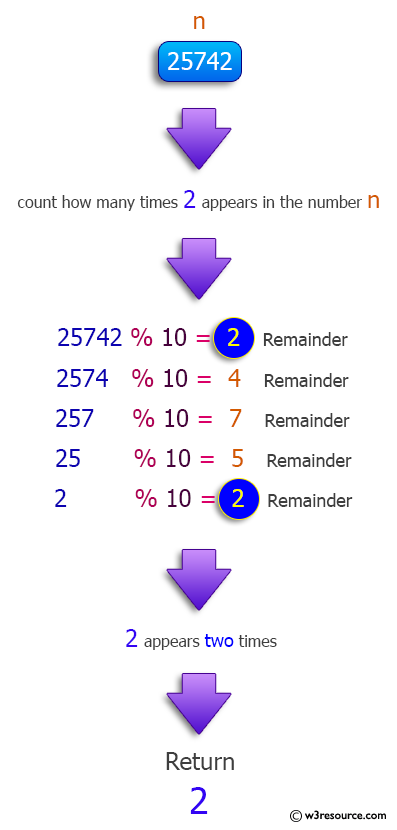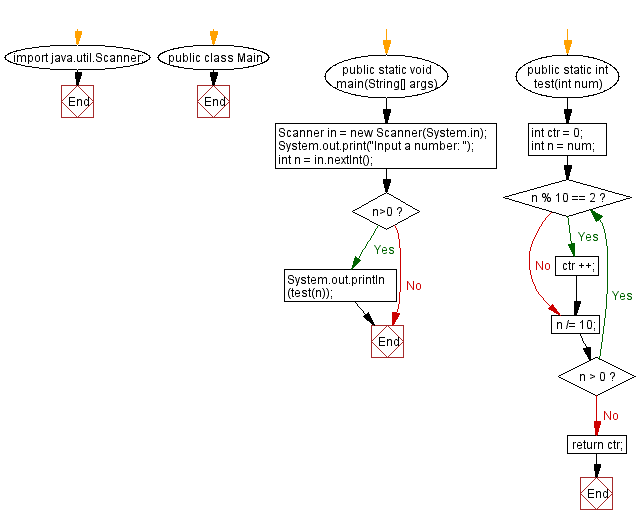﻿ Java: In an integer, count the number of digits with value 2# Java Method Exercises: In an integer, count the number of digits with value 2

## Java Method: Exercise-17 with Solution

Write a Java method to count the number of digits in an integer that have the value 2. The integer may be assumed to be non-negative.

Pictorial Presentation:Sample:
Input: 12541
Output: 1
Input: 25672
Output: 2
Input: 9484
Output: 0

Sample Solution:

Java Code:

``````import java.util.Scanner;
public class Main {
public static void main(String[] args)
{
Scanner in = new Scanner(System.in);
System.out.print("Input a number: ");
int n = in.nextInt();
if (n>0)
{
System.out.println(test(n));
}
}
public static int test(int num)
{
int ctr = 0;
int n = num;
do{
if (n % 10 == 2){
ctr ++;
}
n /= 10;
}while(n > 0);
return ctr;
}
}
```
```

Sample Output:

```Input a number:  12541
1
```

Flowchart :Java Code Editor:

Previous Java Exercise: Find all twin prime numbers less than 100.
Next Java Exercise: Three integers and check whether they are consecutive

What is the difficulty level of this exercise?

Test your Programming skills with w3resource's quiz.

﻿